Published on

# JavaScript Set, Every Junior Should Know

Authors
•Name
Imamuzzaki Abu Salam
@imamdev_

Why Set? It's a data structure that allows you to store unique values of any type. It's similar to an array but doesn't allow duplicate values. It's a great way to hold unique values of any kind. Sometimes you want to store unique values in an array, but you don't want to loop through the Array to check if the value already exists. That's where Set comes in handy.

In a previous article, we discussed Javascript Map. Set is similar to Map, but it only stores the keys, not the values. It's a great way to keep unique values of any type. It's overwhelming to use Map in so many cases. That's why let's talk about Set.

## What is JavaScript Set?

Set objects are collections of values. A value in the Set may only occur once; it is unique in the Set's collection. You can iterate through the elements of a set in insertion order. The insertion order corresponds to the order in which each piece was inserted into the Set by the add() method successfully (that is, there wasn't an identical element already in the Set when Set called add()).

The specification requires sets to be implemented "that, on average, provide access times that are sublinear on the number of elements in the collection." Therefore, it could be represented internally as a hash table (with O(1) lookup), a search tree (with O(log(N)) lookup), or any other data structure, as long as the complexity is better than O(N).

## How to create a Set?

There are two ways to create a Set. The first way is to use the Set constructor. The second way is to use the Set object literal.

### Set constructor

The Set constructor creates a new Set object. You can pass an iterable object as an argument to the constructor. The iterable object's elements will be added to the new Set.

``````const set = new Set([1, 2, 3, 4, 5])
``````

### Set object literal

The Set object literal creates a new Set object. You can pass an iterable object as an argument to the object literal. The iterable object's elements will be added to the new Set.

``````const set = {1, 2, 3, 4, 5};
``````

## Set Methods & Property

MethodDescription
add()Adds a new element with a specified value to the Set object.
clear()Removes all elements from the Set object.
delete()Removes the element associated with the value and returns the value that Set.prototype.has(value) would have previously returned. Set.prototype.has(value) will return false afterward.
entries()Returns a new Iterator object that contains an array of [value, value] for each element in the Set object, in insertion order.
forEach()Executes a provided function once per each value in the Set object, in insertion order.
has()Returns a boolean asserting whether an element is present with the given value in the Set object.
keys()Returns a new Iterator object that contains the values for each element in the Set object in insertion order.
values()Returns a new Iterator object that contains the values for each element in the Set object in insertion order.
PropertyDescription
sizeReturns the number of values in the Set object.

## Set Examples

### Add a value to Set

``````const set = new Set([1, 2, 3, 4, 5])
console.log(Set) // Set { 1, 2, 3, 4, 5, 6 }
``````

### Delete a value from Set

``````const set = new Set([1, 2, 3, 4, 5])
set.delete(3)
console.log(set) // Set { 1, 2, 4, 5 }
``````

### Check if a value exists in Set

``````const set = new Set([1, 2, 3, 4, 5])
console.log(set.has(3)) // true
``````

### Iterate through Set

``````const set = new Set([1, 2, 3, 4, 5])
for (const item of Set) {
console.log(item)
}
// 1
// 2
// 3
// 4
// 5
``````

### Convert Set to Array

``````const set = new Set([1, 2, 3, 4, 5])
const array = Array.from(set)
console.log(array) // [1, 2, 3, 4, 5]
``````

### Convert Array to Set

``````const Array = [1, 2, 3, 4, 5]
const set = new Set(array)
console.log(Set) // Set { 1, 2, 3, 4, 5 }
``````

### Get the size of Set

``````const set = new Set([1, 2, 3, 4, 5])
console.log(set.size) // 5
``````

### Clear Set

``````const set = new Set([1, 2, 3, 4, 5])
set.clear()
console.log(set) // Set {}
``````

### Merge Set

``````const set1 = new Set([1, 2, 3, 4, 5])
const set2 = new Set([6, 7, 8, 9, 10])
const set3 = new Set([...set1, ...set2])
console.log(set3) // Set { 1, 2, 3, 4, 5, 6, 7, 8, 9, 10 }
``````

### Get the intersection of two Sets

``````const set1 = new Set([1, 2, 3, 4, 5])
const set2 = new Set([4, 5, 6, 7, 8])
const set3 = new Set([...set1].filter((x) => set2.has(x)))
console.log(set3) // Set { 4, 5 }
``````

### Get the difference between two Sets

``````const set1 = new Set([1, 2, 3, 4, 5])
const set2 = new Set([4, 5, 6, 7, 8])
const set3 = new Set([...set1].filter((x) => !set2.has(x)))
console.log(set3) // Set { 1, 2, 3 }
``````

### Check if Set is a subset of another Set

``````const set1 = new Set([1, 2, 3, 4, 5])
const set2 = new Set([4, 5])
console.log([...set2].every((x) => set1.has(x))) // true
``````

### Check if Set is a superset of another Set

``````const set1 = new Set([1, 2, 3, 4, 5])
const set2 = new Set([4, 5])
console.log([...set1].every((x) => set2.has(x))) // false
``````

### Check if two Sets are disjoint

``````const set1 = new Set([1, 2, 3, 4, 5])
const set2 = new Set([6, 7, 8, 9, 10])
console.log([...set1].every((x) => !set2.has(x))) // true
``````

### Check if two Sets are equal

``````const set1 = new Set([1, 2, 3, 4, 5])
const set2 = new Set([1, 2, 3, 4, 5])
console.log(set1.size === set2.size && [...set1].every((x) => set2.has(x))) // true
``````

## Browser Support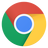Chrome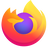Firefox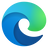Edge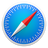Safari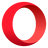Opera
49+44+12+10.1+36+

## Set vs. Array

SetArray
Unique value, disallow duplicate valueAllow duplicate value
Set is a collection of values in no particular orderArray is a collection of values in a particular order
Set is iterableArray is iterable
Set is slower than an array in initialization because it uses a hash process.Array is faster in terms of initialization
Performance is better when checking for the existence of an elementPerformance is better when accessing an element